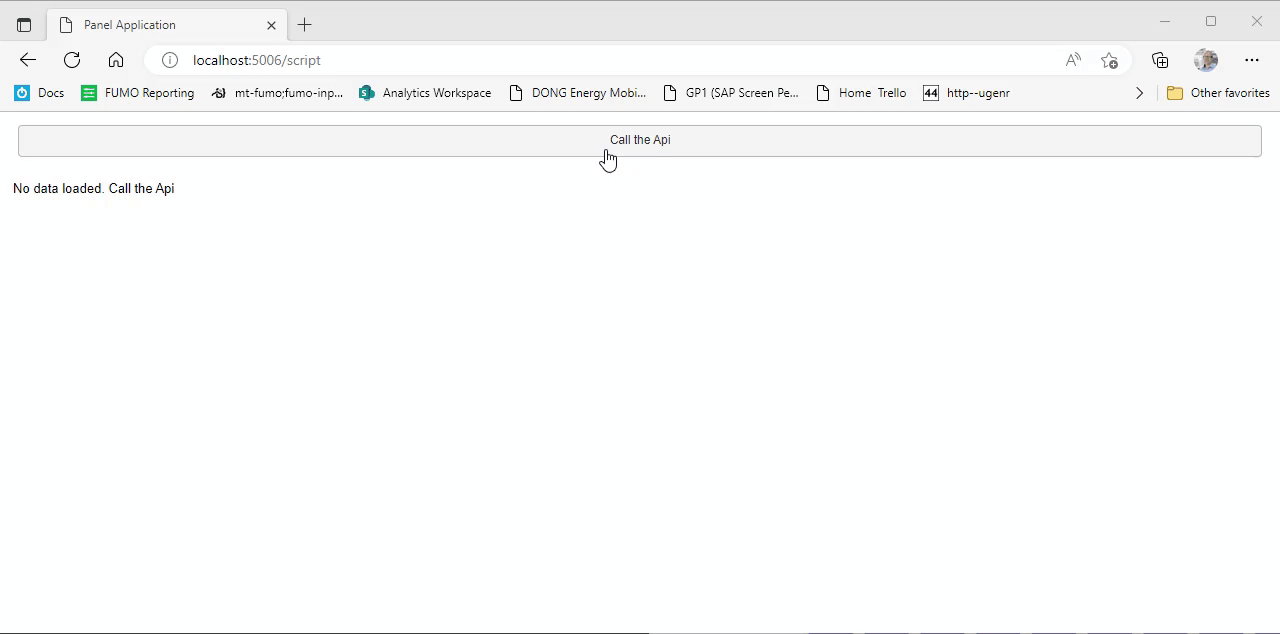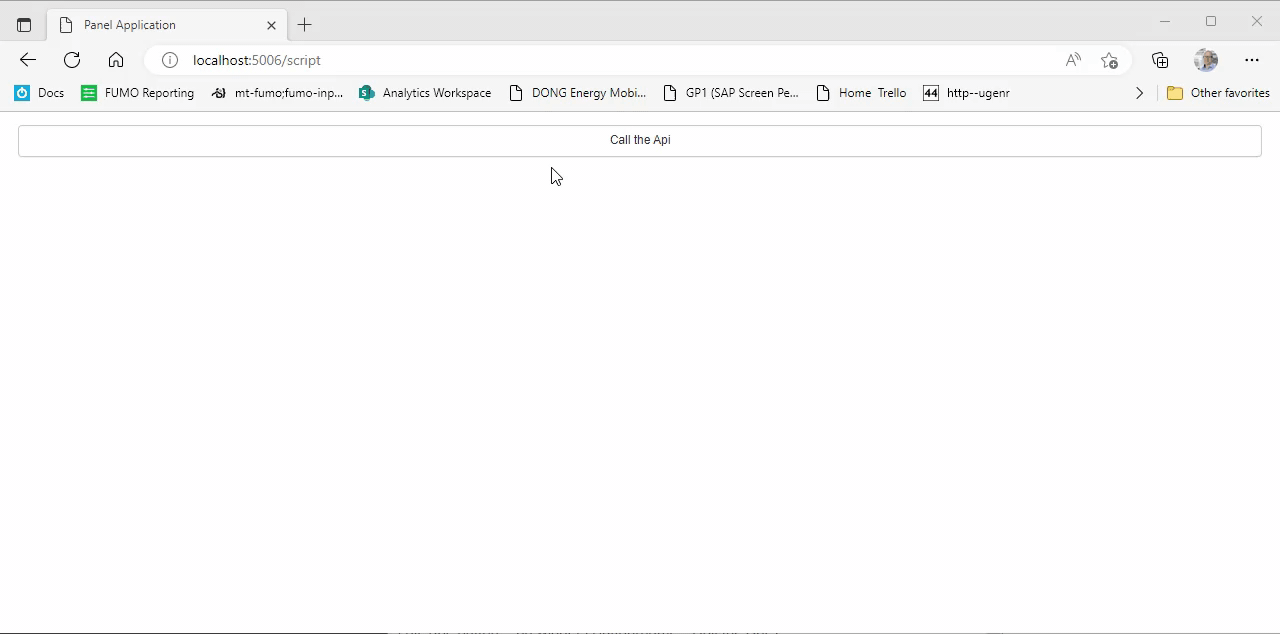# Issue with empty plot (pn.pane.plotly()) Vs. None

Hello Everyone,

## Context:

I have been working on building a dashboard using FastListTemplate, where in I have inputs, button in sidebar and tabs in the main area with plots under each tabs to be displayed to the users based on the inputs selected.

## Background:

To get to understanding my problem I am doing the following:

A: Initialize with empty plot

``````obj_plot = pn.pane.Plotly()
``````

B: Plot generation

``````def distribution_plot(
df,
x_column,
y_columns,
color_names,
title,
xaxis_title,
yaxis_title,
text_format=".0f",
):
df[x_column] = df[x_column].apply(format_bin)
fig = go.Figure()

for i in range(len(y_columns)):
go.Bar(
x=df[x_column],
y=df[y_columns[i]],
name=color_names[i],
text=df[y_columns[i]],
textposition="outside",
marker_color=px.colors.qualitative.Plotly[i],
textfont=dict(size=12),
)
)

fig.update_traces(texttemplate=f"%{{text:{text_format}}}", textposition="outside")
fig.update_layout(
barmode="group",
title=title,
xaxis_title=xaxis_title,
yaxis_title=yaxis_title,
template="plotly_dark",
width=850,
height=500,
)
return fig
``````
``````my_plot = def distribution_plot(....)
obj_plot.object = my_plot
``````

C: API call - display plot

I did A + B + C and it is a success

## Problem:

1. I do not want an empty plot to start with as shown in figure1
2. I want to start off with blank to startwith as shown in figure2

so,
I did
A (obj_plot = None) + B + C and it is a Failure (plot does not get displayed upon api call)

Is there a way to start off with Blank(figure2) and be able to display the plot after the api call ?

Thanks.
p.s. I am relatively new panel user.

Hi @venv,

It’s best to post a minimal example users can paste into their system and run to be able to help. That said the idea is to create for example a pn.column then you can append and remove your graphs to this but the column must be generated on load as I understand

Maybe something like this will help

``````import panel as pn
import numpy as np
import holoviews as hv

pn.extension(sizing_mode = 'stretch_width')

button_append = pn.widgets.Button(name='Append', button_type='primary')
button_pop = pn.widgets.Button(name='Pop', button_type='danger')

def button_append_graph(event):
if (len(column))>=0:
column.append(hv.Scatter([]))

button_append.on_click(button_append_graph)

def button_pop_graph(non):
if len(column)>1:
column.pop(1) #remove 1 plot

button_pop.on_click(button_pop_graph)

column = pn.Column('graph will append here', sizing_mode="stretch_width", height=1000)
pn.Row(pn.Column(button_append,button_pop), column, sizing_mode="stretch_width", height=100)
``````
2 Likes

There are many ways additional to achieve this.

## Plot function that depends on data

``````import panel as pn
import plotly.express as px

pn.extension("plotly", sizing_mode="stretch_width")

# Used to hold the data from the api
data = pn.widgets.DataFrame()

call_api_button = pn.widgets.Button(name="Call the Api")

@pn.depends(call_api_button, watch=True)
def call_api(_):

@pn.depends(value=data)
def plot(value):
if value is None:
return "No data loaded. Call the Api"
else:
return px.bar(value, x='year', y='pop')

pn.Column(
call_api_button, plot,
).servable()
``````## Visibility of Plotly pane depends on its object

``````import panel as pn
import plotly.express as px

pn.extension("plotly", sizing_mode="stretch_width")

plotly_pane = pn.pane.Plotly(visible=False)
call_api_button = pn.widgets.Button(name="Call the Api")

@pn.depends(call_api_button, watch=True)
def call_api(_):
plot = px.bar(data, x='year', y='pop')
plotly_pane.object = plot

@pn.depends(value=plotly_pane.param.object, watch=True)
def plot(value):
if value is None:
plotly_pane.visible=False
else:
plotly_pane.visible=True

pn.Column(
call_api_button, plotly_pane,
).servable()
``````2 Likes

Thanks @Marc for showing me with the example.

1 Like

Thanks @carl for the code and I noted your suggestion as well.

1 Like